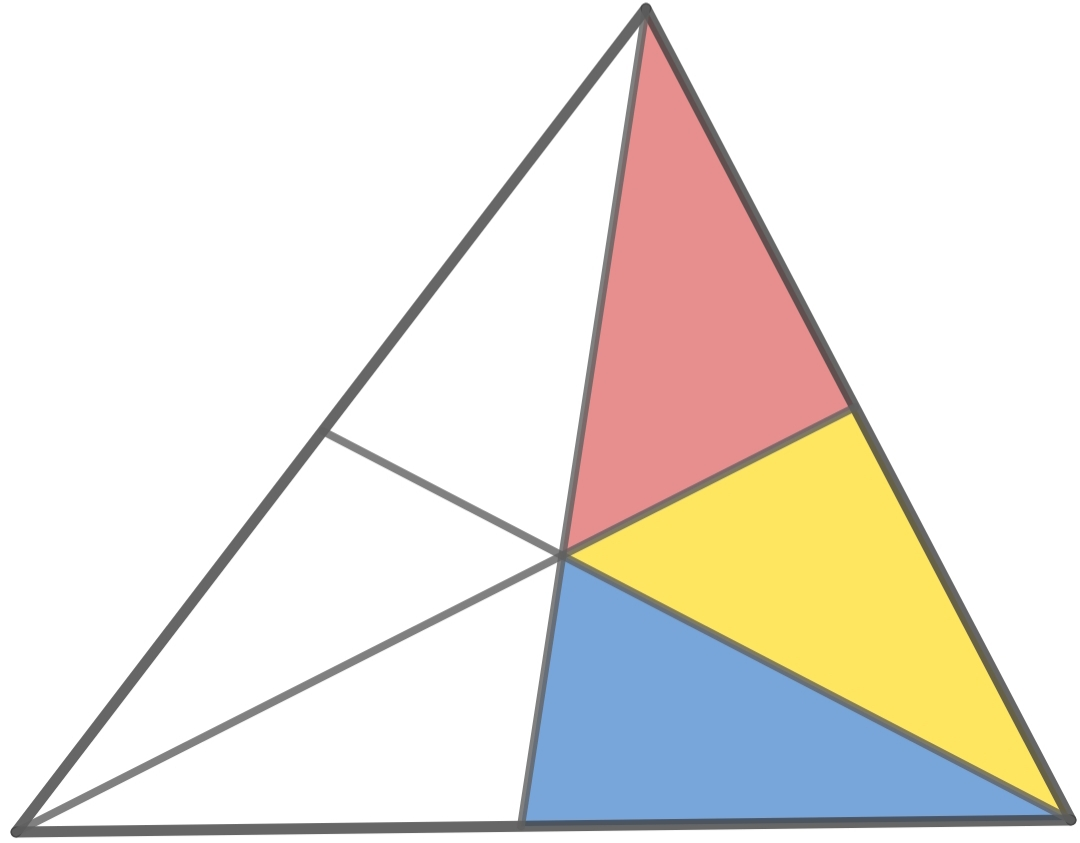# Thread: Minimum area of triangle

1. ## Minimum area of triangleHello,
The figure shows a triangle divided into six regions using line segments drawn from the vertices to the opposite sides.
The red and blue regions have the same areas of 1 unit.
What should be the area of the yellow region so that the area of the whole triangle is minimum?

Solution:- I don't know the solution to this problem. If any member knows the correct answer may reply.2. ## Re: Minimum area of triangle

Are the line segments medians, angle bisectors, or just arbitrarily drawn from each vertex to the side opposite that vertex?

3. ## Re: Minimum area of triangleOriginally Posted by CervesaAre the line segments medians, angle bisectors, or just arbitrarily drawn from each vertex to the side opposite that vertex?
Hello,

They are just lines arbitrarily drawn from the vertices of a triangle.

4. ## Re: Minimum area of trianglearea of triangle $ABC=[ABC]=x+2+a+b+c$

we have

$$\frac{b+c}{x+1}=\frac{a}{1}$$

and

$$\frac{a+1}{b+c}=\frac{x}{1}$$

From this we get

$$\frac{a+1}{x}=a(x+1)$$

Replacing in the equation for the area

$$[ABC]=\frac{x(x+1)(x+2)}{x^2+x-1}$$

#### Search Tags

area of triangle, calculus